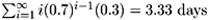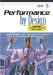# 10.6 Model Interpretation

 Although knowing the steady state probabilities of being in each system state is technically "the solution" of the model, it is not especially helpful unless these probabilities can be properly interpreted and used to answer more practical questions. This is illustrated in the context of the two motivating examples. Random Walk Through England: Model Interpretation In Section 10.3, four motivating questions were posed that can now be answered. Father's question: What percentage of days is the son actually not drinking in Leeds?Answer: 74%. Since the steady state probability of being in state 1 (i.e., drinking in a Leeds pub)is 0.2644 (i.e., 26%), the rest of the time the lad is sightseeing, kayaking, or hiking.Lake District relatives' question: Once the son finishes a day of kayaking in the Lake District, how long will it typically be before he returns?Answer: 3.33 days. The mean time between entering a particular state (i.e., the state's "cycle time") is the inverse of the steady state probability of being in that state. Since the steady state probability of being in state 3 (i.e., kayaking in the Lake District) is 0.2308, the cycle time between successive entries into state 3 is 1/0.2308 = 4.33 days. Since it takes one day for the lad to kayak, the time from when he finishes a day of kayaking until he typically starts kayaking again is 4.33 1 = 3.33 days. (Note: To grasp an intuitive sense of this relationship of cycle time being the inverse of the steady state probability, consider a hypothetical five state model where the lad cyclically visits each of the five states for one day each. Since each of the five states is equivalent, the lad is in each state 20% of his time. Thus, the cycle time between successive entries to each state is 1/0.20 = 5 days and the time between leaving a state until the state is reentered is 5 1 = 4 days.)Policeman's question: How many days each month can the bobbies expect to see the son driving to London after drinking in Leeds?Answer: 4.76 days. Consider a 30 day month. The steady state probability of being found drinking in a Leeds pub (i.e., state 1) on any particular day is 0.2644. Thus, out of 30 days, 30 x 0.2644 = 7.93 days will find the lad drinking. However, since the lad decides to go to London with only probability 0.6 after a day of drinking in Leeds, the bobbys can expect to find the lad on the road to London 7.93 x 0.6 = 4.76 days each month.Kayak renters' question: How many visits each month does the son typically visit their shop and typically how long does the son keep their kayak out each visit?Answer: 2.08 visits per month, keeping the kayak an average of 3.33 days each visit. The only way to enter state 3 (i.e., kayaking) from another state is from state 4 (i.e., hiking). That is, the lad arrives to the Lake District only after hiking in the moors. The steady state probability of being found hiking on any particular day is 0.3462, or 30 x 0.3462 = 10.39 days each month. However, after hiking for a day, the lad only decides to go kayaking the next day with probability 0.2. Therefore, the lad typically starts a new visit to the Lake District 10.39 x 0.2 = 2.08 times each month. This answers the first part of the question. To answer the second part, since the steady state probability of being found kayaking is 0.2308 on any particular day, the lad can be expected to be kayaking 30 x 0.2308 = 6.92 days out of each month. If he makes only 2.08 new visits each month, the duration of each visit is typically= 3.33 days. An alternative, yet equivalent, approach to the solution is to note that the lad kayaks for only one day with probability 0.3. He kayaks for exactly two days with probability 0.7 x 0.3. He kayaks for exactly three days with probability (0.7)2 x 0.3 and, in general, he kayaks for exactly n days with probability (0.7)n 1 x 0.3. The average time spent kayaking per visit is, thus,. (It is only a numerical coincidence in this particular example that the answer to the Lake District relatives' question is the same as the answer to the second part of the kayak renters' question.) Putting aside the analogy for a moment, the first question (i.e., the father's question) relates to the device utilization of the resources within the system being modeled. This is the probability of being in certain system states. The second question (i.e., the Lake District relatives' question) relates to the response time of the system. This is the time that it takes to return to a state after leaving the state. The third and fourth questions (i.e., the policeman's and the kayak renters' questions) relate to system throughput. This is the rate of traffic along certain arcs within the model. Database Server Support: Model Interpretation Also in Section 10.3, four motivating questions were posed relative to the database server example that can now be answered. User's question: What response time can the typical user expect?Answer: 44.24 seconds per user transaction. The response time can be found via application of the Interactive Response Time Law, R = M/X0 Z, introduced in Chapter 3. (See Fig. 3.4) With the think time Z being 0 (i.e., there is no think time component with the submitted user transactions in this example), the response time, R reduces to the simple ratio of the average number of users in the system, M, to the system throughput, X0. In this example, the number of users in the system is fixed at two. The throughput of the system, measured at the CPU, is the product of its utilization and its service rate. The CPU is utilized in states (2,0,0),(1,1,0), and (1,0,1). Knowing the solution to the steady state probabilities of these states, the CPU utilization is P(2,0,0) + P(1,1,0) + P(1,0,1) = 0.1391 + 0.1043 + 0.2087 = 0.4521. The service rate of the CPU is 6 transactions per minute. Therefore, the throughput measured at the CPU is 0.4521 x 6 = 2.7126 transactions per minute, resulting in an average response time of 2/2.7126 = 0.7373 minutes per transaction, which equals 0.7373 x 60 = 44.24 seconds per user transaction.System administrator's question: How near capacity (i.e., what is the utilization) of each of the system resources?Answer: The CPU's utilization is 0.4521, the fast disk's utilization is 0.3391, and the slow disk's utilization is 0.6783. These are found as direct sums of the relevant steady state probabilities. The CPU's utilization was calculated above. The fast disk is utilized in states (1,1,0),(0,2,0), and (0,1,1). Thus, the utilization of the fast disk is P(1,1,0) + P(0,2,0) + P(0,1,1) = 0.1043 + 0.0783 + 0.1565 = 0.3391. In like manner, the utilization of the slow disk is P(1,0,1) + P(0,1,1) + P(0,0,2) = 0.2087 + 0.1565 + 0.3131 = 0.6783.Company president's question: If I can capture Company X's clientele, which will likely double the number of users on my system, I will need to also double the number of active users on my system. What new performance levels should I spin in my speech to the newly acquired customers?Answer: The overall system throughput is predicted to go from 2.7126 transactions per minute to 3.4768 transactions per minute (i.e., up 28%). The user response time, however, is predicted to go from 44.24 seconds per transaction to 69.03 seconds per transaction (i.e., up 56%).These numbers are the result of resolving the model with four active users instead of the original two. The original system had a state space diagram of six states. The new system model has 15 states. The precise model construction, solution, and interpretation is left as an exercise. However, these new performance predictions will help prepare the company president for what to expect.Company pessimist's question: Since I know that the fast disk is about to fail and all the files will need to be moved to the slow disk, what will the new response time be?Answer: 65.00 seconds per transaction (i.e., an increase of 47%). This number is a result of resolving the model with only two devices, the CPU and the slow disk. The resulting state diagram has only three states, one with both users at the CPU, one with a single user at both the CPU and the slow disk, and one with both users at the slow disk. By formulating and solving the balance equations, the resulting steady state probabilities are 0.0769, 0.2308, and 0.6923, respectively. This leads to a CPU utilization of 0.3077 and a system throughput of 1.8462 transactions per minute. By applying the Interactive Response Time Law, a resulting response time of 65.00 seconds per transactions is predicted. By anticipating such effects, the company can prepare for such possibilities. The above two examples demonstrate how to use the knowledge of the steady state probabilities to arrive at more meaningful and more useful performance metrics. As seen, such models can be applied to predict the performance of anticipated new scenarios. Utilization of resources, system throughput, and user response times are the primary performance metrics of interest.Performance by Design: Computer Capacity Planning By Example
ISBN: 0130906735
EAN: 2147483647
Year: 2003
Pages: 166